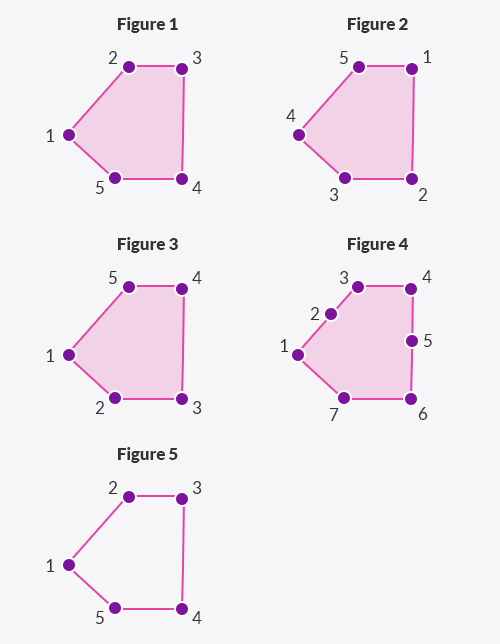Deals Of The Week - hours only!Up to 80% off on all courses and bundles.-Close
Introduction
ST_Equals
5. ST_Equals and equality
Relationships Between Geometries
ST_Distance and ST_Dwithin
Spatial joins practice
Summary

## Instruction

Good job! You may be wondering how the equality operator (=) differs from ST_Equals when comparing geometries. For instance, why didn't we write the query below instead of the original?

SELECT
sf_sights.name
FROM sf_planning_districts
JOIN sf_sights
ON coordinates = boundaries


The equality operator works differently than the ST_Equals function. Moreover, the actual behavior of the equality operator depends on the version of PostGIS you are using. This course uses version 2.3. In PostGIS versions prior to 2.4, the equality operator compared only the bounding boxes of two geometries, ignoring features such as starting points and vertex order. Take a look at the following diagram:Prior to PostGIS 2.4, the equality operator would treat all five figures above as equal, so the linestring would be equal to the polygons.

Since PostGIS 2.4, the behavior of the equality operator (=) has changed. Now, in more recent versions, two geometries are considered equal if they have exactly the same vertices in the same order and with the same starting points. In other words, the equality operator in PostGIS 2.4 considers all five figures above to be different.

In most cases, the kind of equality defined by ST_Equals is the one you want. If you use the equality operator, make sure it really gives the behavior you expected.

## Exercise

Click the button.